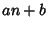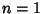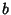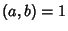## Dirichlet's Theorem

Given an Arithmetic Series of terms, for, 2, ..., the series contains an infinite number of Primes ifandare Relatively Prime, i.e.,. Dirichlet proved this theorem using Dirichlet L-Series.

See also Prime Arithmetic Progression, Prime Patterns Conjecture, Relatively Prime, Sierpinski's Prime Sequence Theorem

References

Courant, R. and Robbins, H. ``Primes in Arithmetical Progressions.'' §1.2b in Supplement to Ch. 1 in What is Mathematics?: An Elementary Approach to Ideas and Methods, 2nd ed. Oxford, England: Oxford University Press, pp. 26-27, 1996.

Shanks, D. Solved and Unsolved Problems in Number Theory, 4th ed. New York: Chelsea, pp. 22-23, 1993.